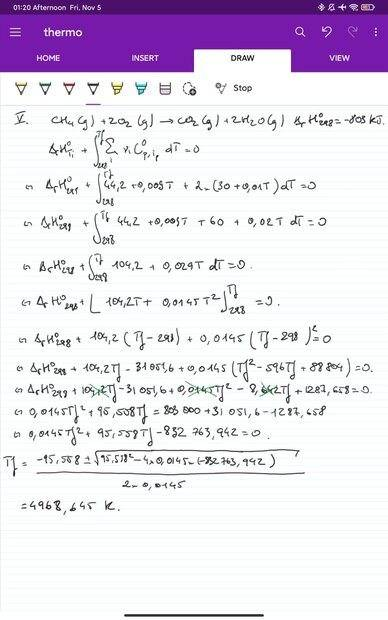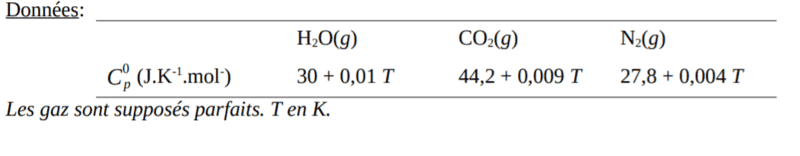# Maximum flame temperature during Combustion of CH4

duchuy
Homework Statement:
Combustion of CH4 at constant pressure (P=1bar).
Relevant Equations:
CH4 (g) + 2O2 (g) --> CO2 (g) + 2H2O (g)
Enthalpy of reaction = -803 kJ.mol-1
Hi, I have to calculate the maximum flame temperature of the reaction above. The answer is 4805K but I didn't manage to obtain that answer. Please help me find my error, thank you so much! The values of C are below the solution attempt.cmb
What does a flame that you can see consist of?

•